# Chemical Reactions Chemical Reactions A process in which

• Slides: 12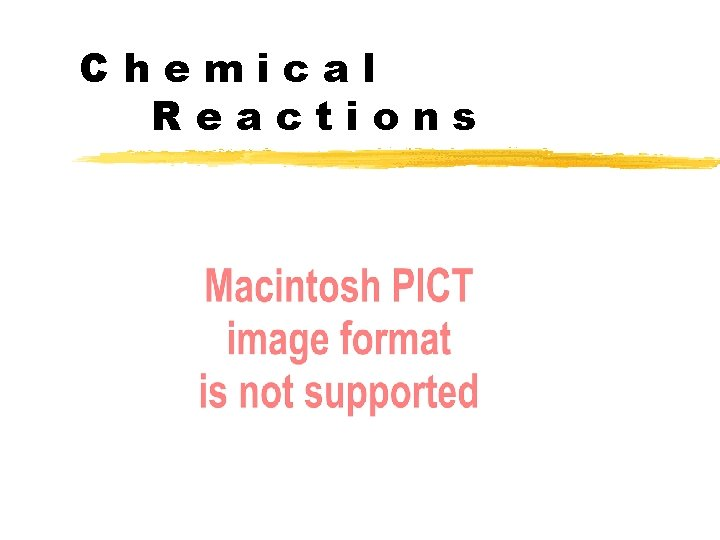Chemical ReactionsChemical Reactions A process in which one substance is changed into another substanceLaw of Conservation of Mass During a chemical r e a c t i o n, a t o m s a r e neither created nor destroyed.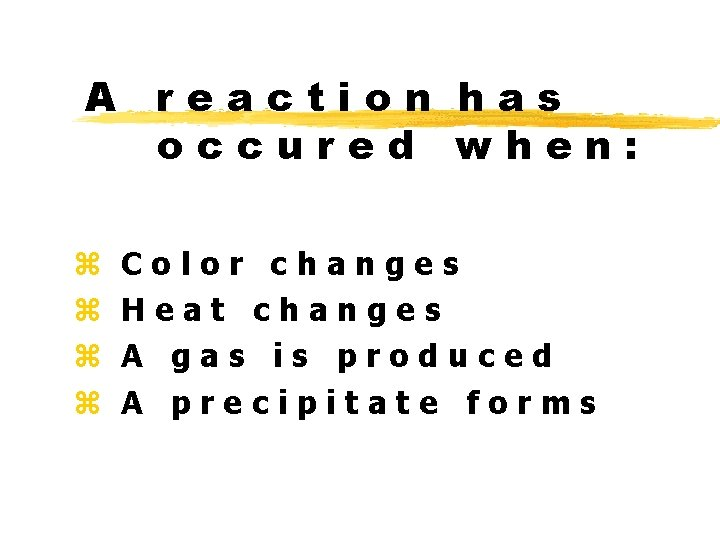A reaction has occured when: z z Color changes Heat changes A gas is produced A precipitate forms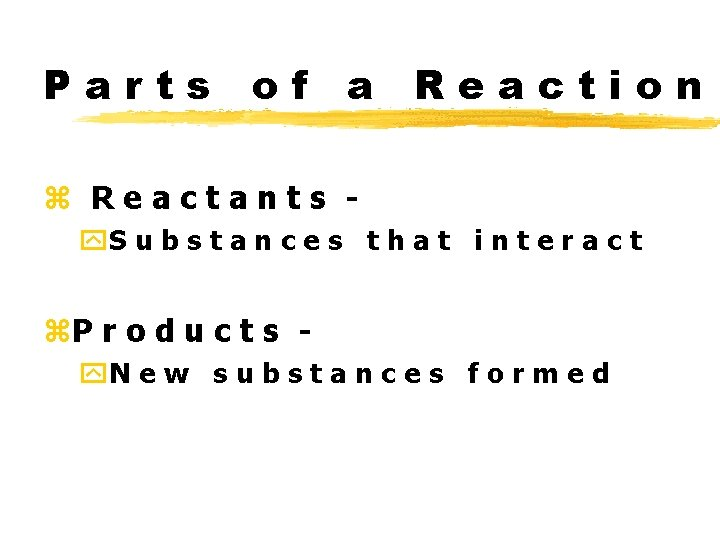Parts of a Reaction z Reactants y. S u b s t a n c e s t h a t i n t e r a c t z. P r o d u c t s y. N e w s u b s t a n c e s f o r m e d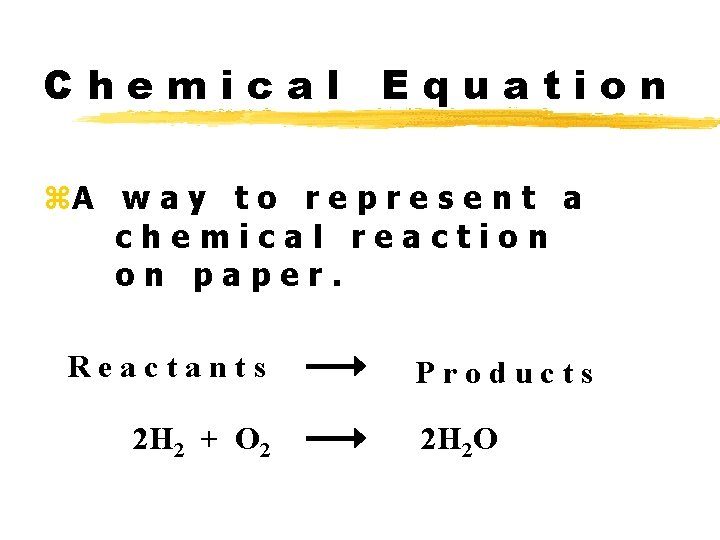Chemical Equation z. A w a y t o r e p r e s e n t a chemical reaction on paper. Reactants 2 H 2 + O 2 Products 2 H 2 O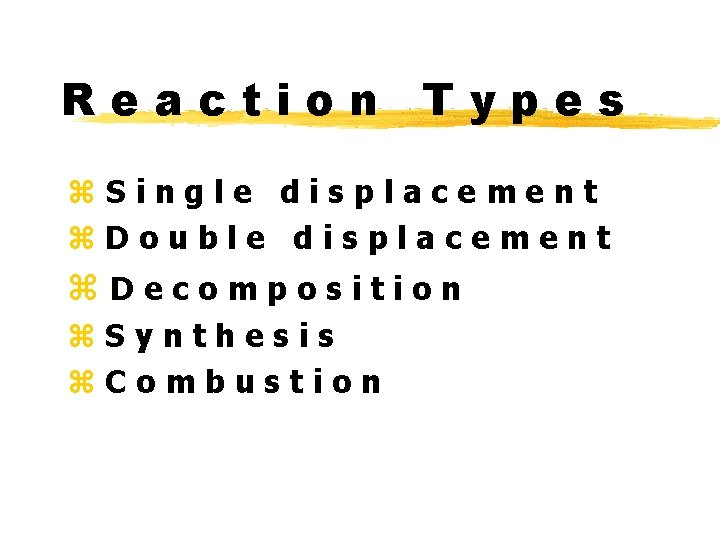Reaction Types z. Single displacement z. Double displacement z. Decomposition z. Synthesis z. Combustion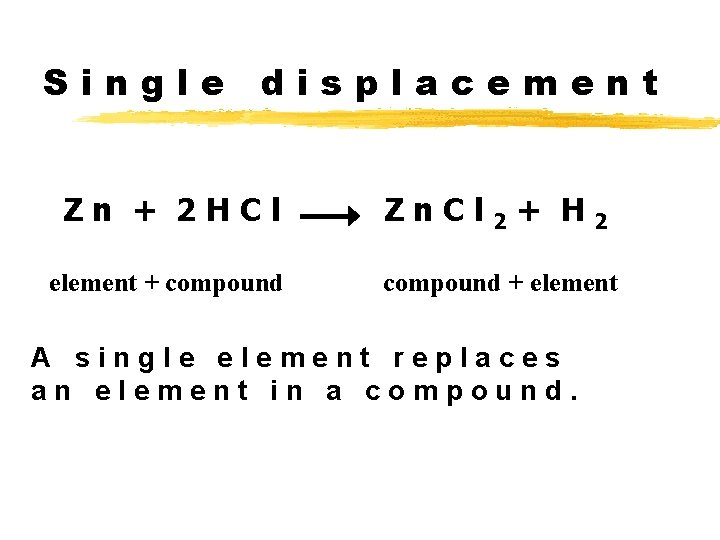Single displacement Zn + 2 HCl element + compound Zn. Cl 2 + H 2 compound + element A single element replaces an element in a compound.Double displacement H 2 SO 4 + 2 Na. OH compound + compound Na 2 SO 4 + 2 H 2 O compound + compound An element from each of two compounds switch places.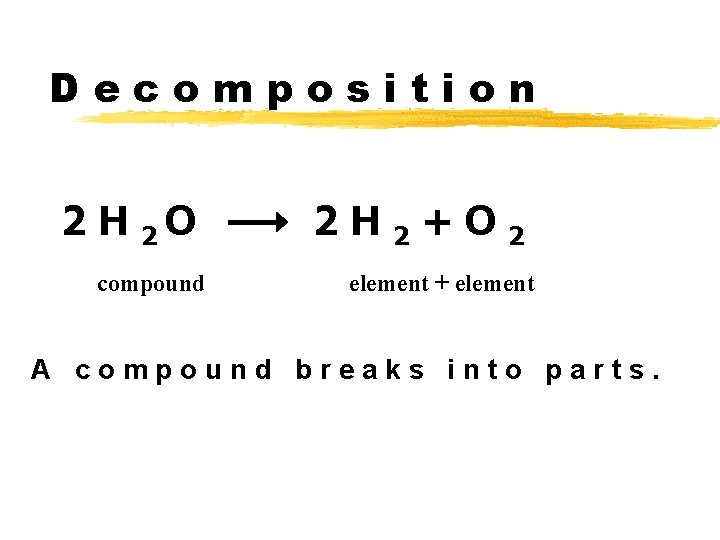Decomposition 2 H 2 O compound 2 H 2+O 2 element + element A compound breaks into parts.Synthesis 2 H 2 + O 2 element + element 2 H 2 O compound Elements or compounds are joined together.Combustion CH 4+ 3 O 2 hydrocarbon + oxygen C O 2 + 2 H 2 O carbon dioxide + water H y d r o c a r b o n s c o m b i n e w i t h o x y g e n, t h e p r o d u c t a a r e a l w a y s CO 2 a n d H 2 O.